## [Tweets]

15/11/2019 6:01am
RT @AandKrobotics: Beyond buzzwords. Our Senior Developer, @l_pitonakova will be talking robots, #AI and #machinelearning and real-world ap…
26/09/2019 7:14am
Day one at #ScirocChallenge: social #robots and humans meet in a shopping mall [LINK]# [V-REP, Gazebo or ARGoS? A Robot Simulators Comparison]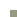Date: Jan 2018

Publication: Pitonakova, L., Giuliani, M., Pipe, A., Winfield, A. (2018) Feature and performance comparison of the V-REP, Gazebo and ARGoS robot simulators. Proceedings of the 19th Towards Autonomous Robotic Systems Conference (TAROS 2018), Lecture Notes in Computer Science, vol 10965, Springer, 357-368.

Disclaimer: This article and the research paper have been written with the use of V-REP 3.5.0, Gazebo 8.1.1. and ARGoS 3.0.0-beta48. Newer versions may vary. Based on work that I have done for the Robotics for Nuclear Environments project at the Bristol Robotics Laboratory (BRL). The article has been published with permission from the BRL.

In agent-based modelling for robotics, it is important to pick a simulation environment that best suits your project. Do you go for speed, model detail, or for a rich set of features? Let’s have a look at three commonly used open-source simulators for robotics: V-REP, Gazebo and ARGoS, to find out which one suits your project the best.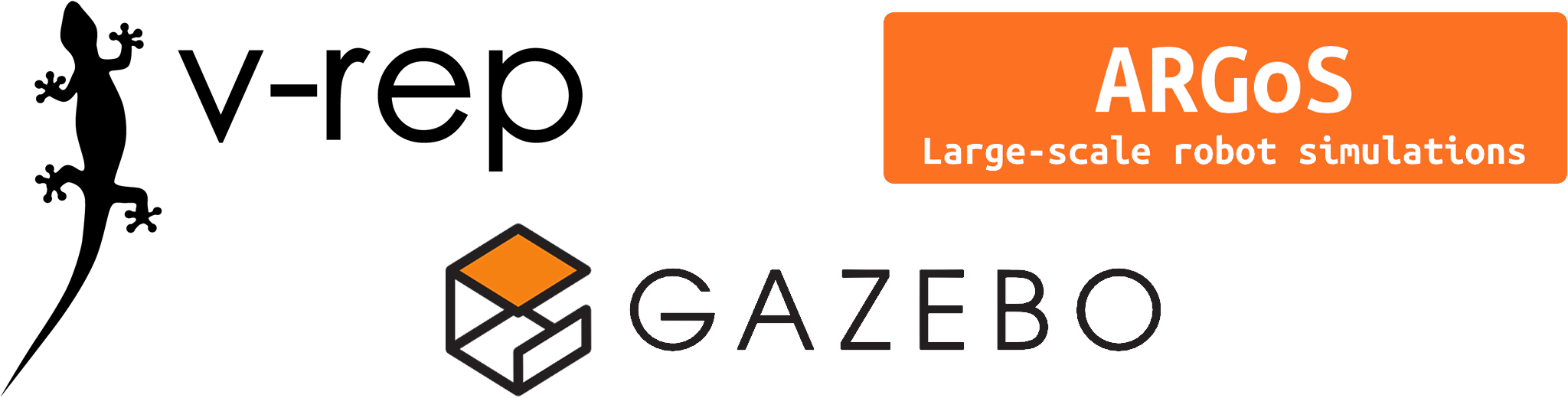• V-REP is a very feature-rich simulation environment that includes a scene and model editor, a large library of models, real-time mesh manipulation, etc. It is available under a commercial licence or for free for educational institutions.
• ARGoS provides a lightweight alternative that is especially relevant for swarm robotics.
• Gazebo represents a middle ground between V-REP and ARGoS. While it can utilise complex 3D meshes and physics engines, it lacks some features that V-REP has, such as mesh manipulation.

### A detailed look at the simulator characteristics

The built-in capabilities, model library, programming methods and user interface of the simulators are compared below. Characteristics with a relative positive impact are marked in green and those with a negative impact in red.

### A detailed look at performance

There were two types of test:

• GUI benchmark involved running a simulator along with its user interface, and attempting to run a 1-minute long simulation in real-time.
• Headless benchmark involved running a simulator from the command line, without its user interface, and attempting to run a 5-minute long simulation as quickly as possible.

There were two types of environment:

• Small scene, where robots were put on a large 2D plane
• Large scene (screenshots below), where an industrial building model withapproximately 41,6000 vertices was imported into the simulator. Since it was not possible to import the model into ARGoS, 5,200 boxes, corresponding to 41,6000 vertices, were randomly placed in the environment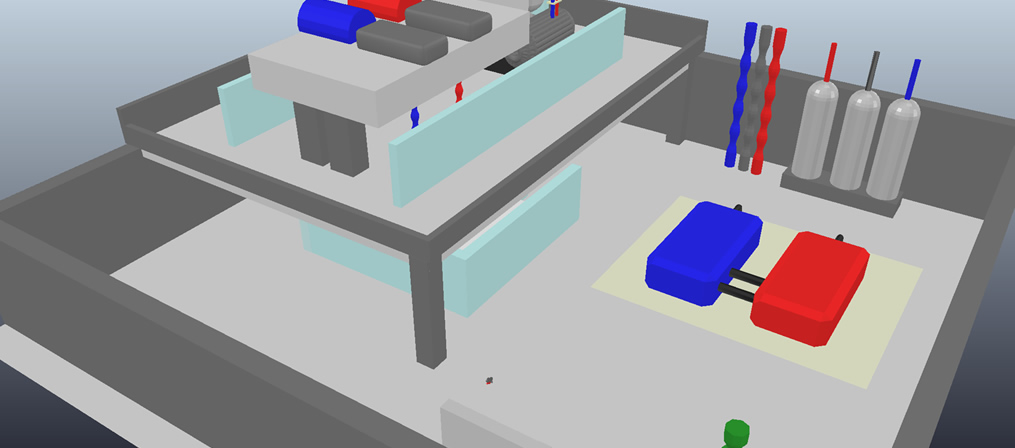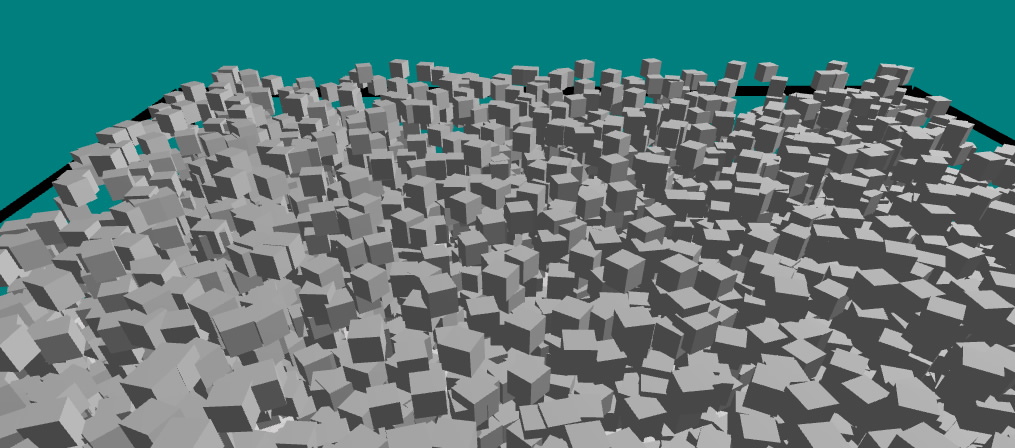The "Large scene" environment in V-REP and Gazebo The "Large scene" environment in ARGoS

Robot models were selected from a library of models available in each simulator, so that a sensible similarity, in terms of robot geometry and controller capabilities, was achieved. In V-REP, the original e-puck model was simplified in order to decrease its vertex count.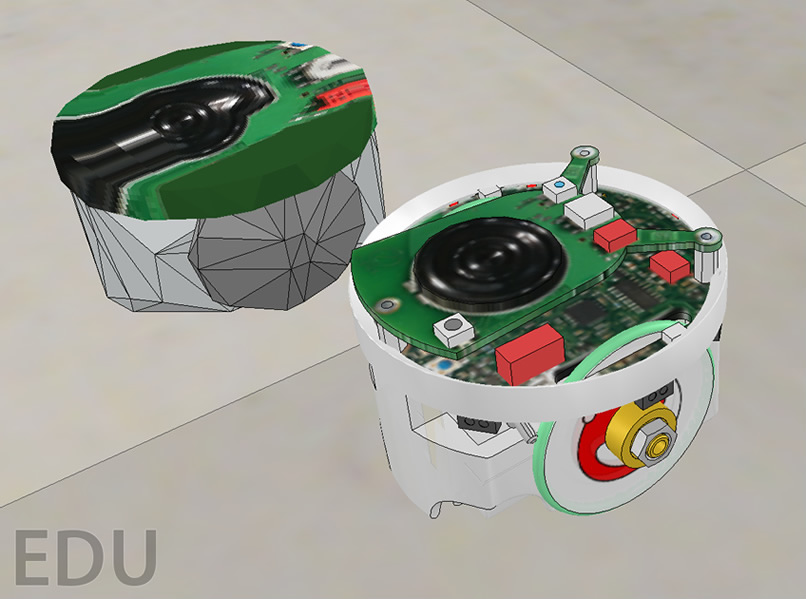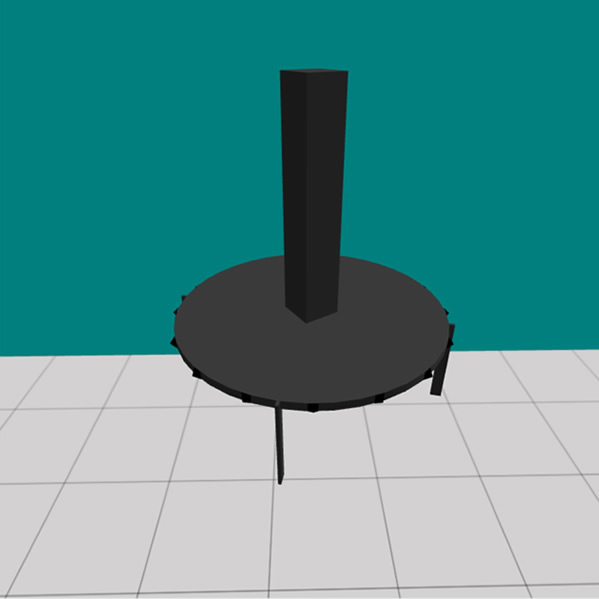A V-REP screenshot of the simplified (left) and original (right) e-puck models A Gazebo screenshot of the Pioneer 3AT robot model An ARGoS screenshot of the eye-bot model

Three performance metrics were used:

• R: Real-time factor, calculated as R = simulated time / real time. When R = 1, the simulation speed corresponded to real time. When R < 1, the simulation ran slower than real time
• C: CPU usage. A usage over 100% indicates that multiple processor cores were used
• M: Memory (RAM) usage.

All tests were performed in a 64-bit Ubuntu Linux 16.04 environment running natively on a MacBook Pro with 4x Intel Core i7 2.2Gz, 8GB RAM and Intel HD Graphics 6000.

Because of long computation times, some experiments were not feasible to run. These cases are marked as “Not feasible” in the tables. Also notice that two values for C and M are reported for Gazebo GUI experiments, corresponding to usage of gzclient (the user interface) and gzserver (the simulator), respectively.

Simulator performance in the GUI mode, with the best (green) and the worst (red) performance indicated:

 V-REP +Bullet 2.78 V-REP +ODE Gazebo +ODE ARGoS +PointMass3D 1 robot +Small scene R ≥ 1C = 180%M = 235 MB R ≥ 1C = 190%M = 225 MB R ≥ 1C = 104+9%M = 225+58 MB R ≥ 1C = 7%M = 85 MB 5 robots +Small scene R = 0.52C = 395%M = 380 MB R = 0.37C = 395%M = 360 MB R ≥ 1C = 105+19%M = 305+58 MB R ≥ 1C = 10%M = 88 MB 10 robots +Small scene R = 0.11C = 400%M = 536 MB R =0.099C = 400%M = 530 MB R ≥ 1C = 105+30%M = 402+58 MB R ≥ 1C = 13%M = 89 MB 50 robots +Small scene Not feasible Not feasible R = 0.87C = 109+105%M = 1410+358 MB R = 0.9C = 103%M = 93 MB 1 robot +Large scene R = 0.96C = 205%M = 235 MB R = 0.53C = 200%M = 225 MB R ≥ 1C = 104+10%M = 264+58 MB R ≥ 1C = 32%M = 90 MB 5 robots +Large scene R = 0.18C = 400%M = 325 MB R = 0.1C = 400%M = 310 MB R ≥ 1C = 105+25%M = 333+58 MB R ≥ 1C = 60%M = 97 MB 10 robots +Large scene R = 0.052C = 400%M = 433 MB R = 0.036C = 400%M = 460 MB R ≥ 1C = 105+40%M = 425+58 MB R = 0.86C = 120%M = 97 MB 50 robots +Large scene Not feasible Not feasible R = 0.57C = 109+105%M = 1450+426 MB R = 0.052C = 107%M = 106 MB

Simulator performance in the Headless mode, with the best (green) and the worst (red) performance indicated:

 V-REP +Bullet 2.78 V-REP +ODE Gazebo +ODE ARGoS +PointMass3D 1 robot +Small scene R = 4.1C = 200%M = 165 MB R = 3.12C = 200%M = 160 MB R = 42.85C = 103%M = 107 MB R = 300C = 6.3%M = 18 MB 5 robots +Small scene R = 0.38C = 400%M = 320 MB R = 0.32C = 400%M = 320 MB R = 10C = 103%M = 130 MB R = 150C = 100%M = 20 MB 10 robots +Small scene R = 0.09C = 400%M = 470 MB R = 0.08C = 400%M = 480 MB R = 5.26C = 103%M = 150 MB R = 21.42C = 144%M = 20 MB 50 robots +Small scene Not feasible Not feasible R = 1.06C = 103%M = 356 MB R = 0.52C = 103%M = 25 MB 1 robot +Large scene R = 1.91C = 200%M = 165 MB R = 0.58C = 200%M = 160 MB R = 18.75C = 103%M = 174 MB R = 15.78C = 139%M = 31 MB 5 robots +Large scene R = 0.2C = 400%M = 270 MB R = 0.11C = 400%M = 250 MB R = 5.88C = 103%M = 192 MB R = 5.45C = 157%M = 45 MB 10 robots +Large scene Not feasible Not feasible R = 3.09C = 103%M = 211 MB R = 1.59C = 130%M = 47 MB 50 robots +Large scene Not feasible Not feasible R = 0.60C = 103%M = 423 MB R = 0.03C = 105%M = 55 MB

### The verdictV-REP ﻿is the most complex and the most resource-hungry of the three simulators. However, it offers a number of useful features, such as multiple physics engines, a comprehensive model library, the ability of a user to interact with the world during simulation and, most importantly, mesh manipulation and optimisation. Moreover, V-REP automatically spawns new threads on multiple CPU cores and therefore utilises the full amount of CPU power when it is necessary. Gazebo failed to exhibit this ability and some problems were noted in ARGoS1 during the experiments performed here. V-REP is suitable for high-precision modelling of robotic applications such as object transportation or area surveillance, as well as of various industrial applications, where only a few robots are required to operate at the same time.ARGoS ﻿on the other hand, is a suitable choice for simulations of swarm robotics tasks, such as collective foraging, flocking, or area coverage. Compared to V-REP, ARGoS trades-off robot, environment and physics complexity for superior performance. An XML-based simulation settings file is also very convenient, especially when a large variety of simulations need to be generated automatically. However, there are multiple important features missing from ARGoS, most notably the ability to import 3D meshes into the simulator. Currently, users that are not willing to spend time and effort on programming new robot models in OpenGL, have fairly limited choices.Gazebo ﻿occupies the space between V-REP and ARGoS. While it is much closer to V-REP in terms of features, its interface and default robot models are much simpler and resemble those found in ARGoS. It is notable that Gazebo outperformed ARGoS in the larger simulation environments studied here, which suggests that it is a more suitable choice for large swarm robotics experiments. However, our experiments showed that the usability of Gazebo is relatively poor. Firstly, while it can import 3D meshes, there are no editing options, making it difficult to alter and optimise models. Another problem is the interface that has a number of issues and fails to follow established conventions. Finally, difficulties were noted when installing dependencies for Gazebo and for many of its third-party models. While not necessarily severe by themselves, these issues together could have a negative impact on a research project.

Note 1: ARGoS does support multi-threading, however, it had problems with spawning threads in experiments with a large number of robots, making the CPU usage increasingly smaller with an increasing number of robots. This problem occurred in both MacOS and Linux environments.

Edits on 18/Jan/2018:
Thanks to Giovanni Beltrame and Carlo Pinciroli for alerting me to some features of ARGoS, such as the ability to interact with robots, create custom UI widgets and using Kilobots. The text has been changed to reflect this. Also, thanks to Giovanni for pointing out that multi-threading in ARGoS should work. Since it worked well for swarms of up to 50 robots, the text has been changed to reflect this. Also, the performance of ARGoS was re-tested with multi-threading on and the numbers in the results tables have been changed.

Edits on 16/Jul/2018:
Information about the conference publication has been added and some text has been changed to match the final version of the paper.

Lenka
[19/05/2019]

Hi John, thanks for your comment. Could you please elaborate a bit so that others get more detail? What is it that you don't like about Gazebo in particular? And what version are you currently using? Thanks!
John Dickinson
[18/05/2019]

Gazebo blows. No matter how many times I come back to it I always come away disappointed. If you are going to pay the computational penalty you may as well go with Webots instead.
Lenka
[08/11/2018]

Hi Ali, thanks for your question. I have not tried this myself, maybe it would be worth asking on the V-REP forums? http://www.forum.coppeliarobotics.com Please do let us know what you find out!
Lenka
[08/11/2018]

Thanks for the link Jannik, unfortunately I currently do not have time to test whether their answers were correct. However, I definitely should add into the text which version of Gazebo I was testing this on - it may be that things have changed since then.
Ali
[07/11/2018]
Hello,
My question is, if it's possible to export the simulation codes from v-rep to a real robot like Khepera IV
thank you

#### Robot swarms in action

Watch e-puck robots collect resources and bring them back to base. While the previous simulation work helped us to learn a lot about the advantages and disadvantages of communication in swarms, doing similar experiments with real robots is already revealing interesting new things.

#### How to compile code for e-puck robots on your computer

Compiling code natively on e-puck robots or cross-compiling on your computer can be very tedious. Luckily, there is a third option: compiling code on a virtualised robot system on your computer, then sending the program onto the robot.

#### pyCreeper

The main purpose of pyCreeper is to wrap tens of lines of python code, required to produce graphs that look good for a publication, into functions. It takes away your need to understand various quirks of matplotlib and gives you back ready-to-use and well-documented code.

#### Novelty detection with robots using the Grow-When-Required Neural Network

The Grow-When-Required Neural Network implementation in simulated robot experiments using the ARGoS robot simulator.

#### Fast Data Analysis Using C++ and Python

C++ code that processes data and makes it available to Python, significantly improving the execution speed.

#### Designing Robot Swarms

This project looks at the challenges involved in modeling, understanding and designing of multi-robot systems.